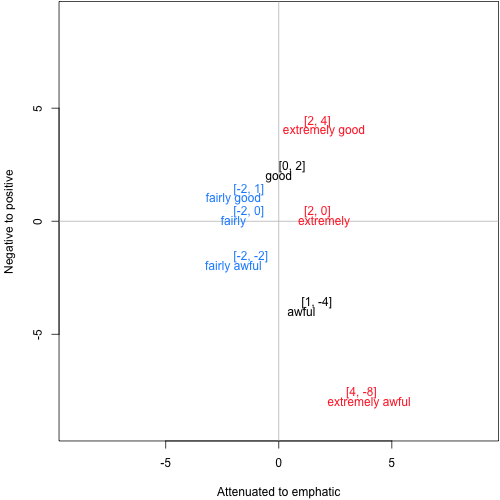HOMEWORK 4

Background

The following depicts a simple, idealized two-dimensional vector-space model in which the x-axis captures emphasis/attenuation and the y-axis captures positive/negative polarity. Each word or phrase is annotated with its vector representation (for example, the vector for good is [0,2]).Question 1 [2 points]

In a sentence or two, describe in informal terms how extremely acts on its argument with respect to the dimensions of emphasis/attenuation and positive/negative.

Question 2 [2 points]

In a sentence or two, describe in informal terms how fairly acts on its argument with respect to the dimensions of emphasis/attenuation and positive/negative.

Question 3 [2 points]

Find matrices $$A$$ and $$B$$ such that the following composition function perfectly predicts the vectors for extremely good and extremely awful:

$p = A a + B b$

Here, $$a$$ is the vector representations of the left word, $$b$$ is the vector representations of the right word, and $$p$$ is the predicted parent vector. (Also: $$Xx$$ is standard matrix multiplication of $$X$$ and $$x$$; and, where $$x$$ and $$y$$ are vectors of dimension $$n$$, $$x + y$$ is the vector $$[ x_{i}+y_{i} , \ldots, x_{n}+y_{n} ]$$.)

Question 4 [2 points]

Assume the $$A$$ and $$B$$ you created in the previous problem are global values used for all adverb–adjective combinations. Apply them to the task of predicting values for fairly good and fairly awful. What values do you obtain? In a sentence or two, articulate what went wrong, perhaps drawing on the insights you expressed in questions 1 and 2.

Question 5 [2 points]

Consider the alternative composition function

$p = Ab$

where $$A$$ is a 2-d matrix representation of the adverb and $$b$$ is a vector representation of its adjectival argument. This approach has the potential to avoid the problem we saw above with the global values $$A$$ and $$B$$. Your task: find a matrix for extremely that perfectly models extremely good and extremely awful.